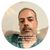# App Development

Announcements
QlikWorld online is next week! REGISTER NOW
cancel
Showing results for
Did you mean:Contributor III

## IF works but Set analysis does not work??

Hi

I have below expression in my app where i have used IF:

Sum(distinct If(If([Booking Amount] > \$(vTopDecile) ,'Y','N')='Y',[Booking Amount],0))

The variable vTopDecile is defined as

fractile(total Aggr(Sum([Booking Amount]),[Opportunity Number],Segment), 0.9)

Now I am trying to write the same expression (the 1st one) using set analysis but that is not working. It always returns 0:

Sum({<[Booking Amount] = {"> \$(=vTopDecile)"}>}[Booking Amount])

Can anybody tell me why?

Is it because fractile cannot work with Set Analysis?

5 RepliesMVP

Can you try this (Make sure you don't have space between > and \$(

Sum({<[Booking Amount] = {">\$(=vTopDecile)"}>}[Booking Amount])

or this

Sum({<[Booking Amount] = {">\$(=\$(vTopDecile))"}>}[Booking Amount])Specialist II

It could be is Segment changes for each record. Set Analysis is evaluated once per chart/visualization, not row for row.Contributor III
Author

1st expression still give 0 but the 2nd one returns value which is wrong.MVP

Your IF has distinct in the aggregation, may be thats making difference -

Sum(distinct {<[Booking Amount] = {">\$(=\$(vTopDecile))"}>}[Booking Amount])MVP

Would you be able to share a sample to look at this?